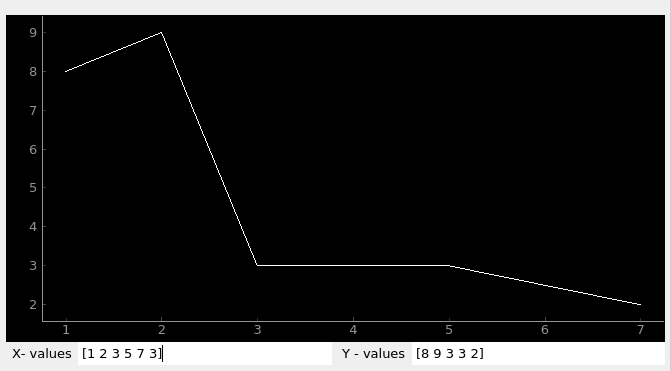# Local Plugin

PyDM uses Data Plugins as sources of information to be displayed at the widgets. Local Data Plugin allows users to create and use local variables.

The Local Data Plugin stores the data that is sent by the widgets through a channel, and broadcasts it to all the listeners connected to this particular local variable channel.

By default, as soon as any widgets gets connected to the same channel variable, they will get the initial values that were defined in the channel’s address. These widgets can receive any updates when the data changes, or they can send data back. For example, if the user has added a Local Variable channel to a PyDMEditLine, then all the widgets connected to this Local Variable will get the values that are updated by the user in the PyDMEditLine.

General Local Plugin channel syntax:

```loc://my_variable_name?type=variable_type&init=initial_values
```

Note

Once a Local Variable channel is created, multiple widgets can be connected to the same channel by providing the name of the variable, like so:

```loc://my_variable_name
```

## Required Attributes

In order to be able to properly create a Local Variable channel, all the required attributes must be provided in the channel’s address.

The table below explains the attributes that are required to create a local plugin channel:

Attributes

Description

Format Example

loc

protocol name for Local Data Plugin

loc://

name

the identifier for a local variable
user’s choice

my_ndarray_var

type

data-type for this variable

type=array

init

initial values to be used

init=[1,2,3,4]

Here is a simple example of a channel address format with the required attributes:

```loc://my_np.array?type=array&init=[1,2,3,4]
```

Note

## Extra Attributes

Along with the required attributes, the Local Data Plugin can also accept some optional attributes to configure the Local Variables with. The optional attributes are described in the extra attributes table below:

The table below explains the optional attributes that can go in the extras:

Attributes

Description

Type

Format Example

precision

precision of float values

int

precision=3

unit

units for the data

string

unit=V

upper_limit

upper control value limit

float or int

upper_limit=100

lower_limit

lower control value limit

float or int

lower_limit=-100

enum_string

new list of values

tuple

enum_string=[‘hey’, ‘hello’]

Note

All the additional attributes are optional, any number of desired attributes can be specified, or none.

Here is a simple example of a channel address format with some optional attributes:

```loc://my.float?type=float&init=1&extras=True&precision=3&unit=V
```

## Variable Types

Local Data Plugin supports the following types:

• int

• float

• bool

• str

• array - this will be treated as a numpy.ndarray type

### Arrays

Note

Arrays will be constructed using numpy.array built in function. See numpy.array for more information on how to construct an array.

The following option must be specified in the “type” value in the channel address:

• type=array

The following extra attributes can be specified. These attributes will be passed in the numpy.array function as parameters when creating the numpy.ndarray. If no attributes are specified, the numpy.array function will use the default values to create a numpy.ndarray.

• Extra Attributes for numpy.array:

Attributes

Description

Type

Format Example

dtype

desired data-type for the array

np.dtype

dtype=float64
dtype=uint8

copy

if True then the object is copied

bool

copy=true (default)

order

memory layout of the array

string

order=K (default)
others {‘A’, ‘C’, ‘F’}

subok

if True then sub-classes
will be passed-through

bool

subok=false (default)

ndmin

minimum number of dimensions

int

ndmin=0 (default)

Here is a simple example with array + extras:

```loc://my_ndarray?type=array&init=[1,2,3,2]&extras=True&dtype=float64&copy=False&order=C&ndmin=0&subok=true
```

## Simple Local Data Plugin Example

The picture below represents a simple example using the Local Data Plugin, where a Waveform Curve Editor has two local data plugin channels:

```loc://y?type=array&init=[1,2,3,4,5,6]&extras=True&dtype=float64

loc://x?type=array&init=[1,2,3,4,5,6]&extras=True&dtype=float64
```

Right below the Waveform Curve Editor widget, there are two other widgets connected to the ‘x’ and ‘y’ local variable respectively:

```X-values: loc://x
Y-values: loc://y
```

Data can be updated in the two X and Y-values widgets and the Waveform Curve Editor will receive the new data and change the curve accordingly, like seen in the picture below:

Waveform Curve Example with ndarrays for X and Y values## Miscellaneous

• If precision is not sent through the “extras”, and it is set to receive the precision from the PV (Process Variable), the Local Data Plugin will match the precision from the values inserted by the users in the widgets.